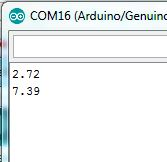# Exponential function

guys.. i want to make exponential function in my program but i couldn't find the example any where how to write it.. for example

You mean the standard c exp()?

``````void setup() {
// put your setup code here, to run once:
Serial.begin(9600);
Serial.println(exp(1));
Serial.println(exp(2));
}

void loop() {
// put your main code here, to run repeatedly:
}
``````

does it have the same value with e^1 and ^2?? i've found the exp () actually but still doubt it because i don't have any arduino at home just using simulator.

exp(), pow(), log(), log10... they are all available.

Perhaps you need pow() ?
https://www.arduino.cc/en/Reference/Pow

These are the functions in the avr gcc library: avr-libc: <math.h>: Mathematics

There are many troubles with simulators, for example with hardware sensors. Using a real Arduino is more fun. Pure mathematical code can be tested in linux and Windows in the normal way.

The '^' operator is used for binary XOR, therefor you have to use the pow() function, because the '^' does not exist for float values.

Output....@Koepel so if i want to make x^2 just wrote x*pow(2)?
@JimboZA thanks a lot man. it has the same value like my calculator.auliawcksn:
it has the same value like my calculator.

You seem surprised....

I gave you a link to pow(), click on it and read the reference : pow(base, exponent)
2x is pow(2, x)

Is your base really the binary number two ? Perhaps you don't need to use float at all ?

@JimboZA what can i say. i'm newbie. haha
@Koepel sorry i don't get what you ask. can use simple english?

Link to the reference of pow() : https://www.arduino.cc/en/Reference/Pow

If you only use '2' as base : 24, 29, 23, then the number '2' is always the base. That is not a floating point calculation, because only binary numbers are used. Then you probably don't need 'float' variables, only a bit shift.

This is floating point: 3.451.23, 4.90.12, and so on. Then you need the pow() function.

Please tell us what your project is, and what you want to calculate.
Your question might be the famous xy-problem: http://xyproblem.info/

To avoid confustion with the XOR operation, it might be better if you all started using superscripts@Koepel actually i have to put this function y=0.0562e^(1.354x) into my program. but i confused how to write the exponent on my program which is already answered. so i guess the base on my function is e.

@AWOL what is super script?? sorry too much ask.i've started programming a month ago so there still many confusing word for me. haha
@Koepel i'm really sorry though i've read it before and didn't understand because they didn't mention the example and i don't what is base and . but now i understand. thanks a lot manOn the editor bar, there's an icon "x2".
Click on it, and you get superscript tags, like this `[sup][/sup]`.
Anything you put between those tags will be displayed as a superscript.

So, to display "x cubed", "x`[sup]3[/sup]`", will show as x3

(In contrast, in C, "2^2" is zero, "2^3" is one, and "2^4" is six.)

That is the natural exponential.
The 'base' number is 2.71828..., but the exp() function is already the natural exponential.

The Arduino has 'e' defined as 'M_E'.
These are the same:

``````y = 0.0562 * exp( 1.354 * x );  // natural exponent
z = 0.0562 * pow( M_E, 1.354 * x );   // same thing
``````

However, no one use a 'pow()' when a natural exponent is used, so the next example uses the 'exp()'.

``````// https://forum.arduino.cc/index.php?topic=387592.0
// Exponential function, with natural exponent.
// Open the Serial Plotter (Ctrl+Shift+L) to see the result in a graph.

void setup()
{
Serial.begin( 9600);
while( !Serial);       // For Leonardo, wait for serial monitor

float x,y;

for( x = 0.1; x < 10.0; x += 0.1)
{
y = 0.0562 * exp( 1.354 * x );
Serial.println( y);
}
}

void loop()
{
}
``````

ohh i see. thanks..

auliawcksn:
@Koepel actually i have to put this function y=0.0562e^(1.354x) into my program.

``````double y = 0.0562 * exp(1.354*x);
``````